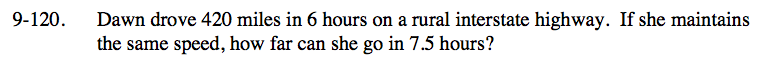### Home > CC3 > Chapter 9 > Lesson 9.2.4 > Problem9-120

9-120.

Dawn drove 420 miles in 6 hours on a rural interstate highway. If she maintains the same speed, how far can she go in 7.5 hours? Homework Help ✎Since Dawn will maintain the same speed, we can find the equivalent fraction for 7.5 hours to solve for the distance.

$\frac{\text{miles}}{\text{hour}} = \frac{420}{6} = \frac{\it x}{7.5}$

$70 = \frac{\it x}{70}$

x = 525 miles